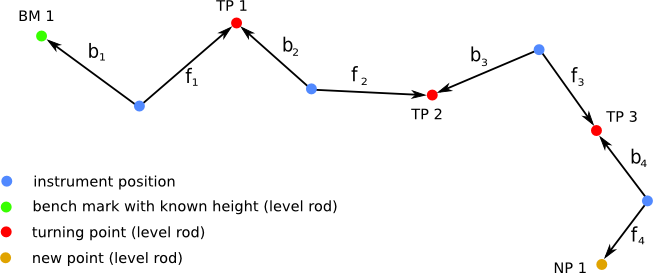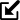# Differential levelling online calculation

 Line and area levelling Example 1 calculates a simple line levelling. Points are measured along a route by continuously moving the surveyor's level device, while each turning point is taken twice (in foresight and backsight). As a first step, enter the number of measuring points into the calculation script. It will determine the number of rows of the main table, which will appear after hitting "enter" or by clicking the "Next"-button. Then - starting from a benchmark (vertical control point with known elevation) - the foresights (f) and backsights (b) need to be entered. In Example 1 potential reading errors cannot be identified, because only one benchmark is specified. Therefore, only the vertical difference to the previous point (rise / fall) and the reduced level of each point are calculated. In practice, this measurement must be checked by a second measurement.Example 1